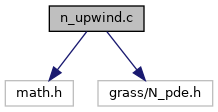GRASS GIS 7 Programmer's Manual  7.9.dev(2021)-e5379bbd7
n_upwind.c File Reference
`#include <math.h>`
`#include <grass/N_pde.h>`
Include dependency graph for n_upwind.c:Go to the source code of this file.

## Functions

double N_full_upwinding (double sprod, double distance, double D)
full upwinding stabilization algorithm More...

double N_exp_upwinding (double sprod, double distance, double D)
exponential upwinding stabilization algorithm More...

## ◆ N_exp_upwinding()

 double N_exp_upwinding ( double sprod, double distance, double D )

exponential upwinding stabilization algorithm

The arguments are values to compute the local peclet number

Parameters
 sprod double – the scalar produkt between the velocity vector and the normal vector between two points distance double – distance between two points D double – diffusion/dispersion tensor part between two points
Returns
the weighting factor

Definition at line 63 of file n_upwind.c.

References D.

## ◆ N_full_upwinding()

 double N_full_upwinding ( double sprod, double distance, double D )

full upwinding stabilization algorithm

The arguments are values to compute the local peclet number

Parameters
 sprod double – the scalar produkt between the velocity vector and the normal vector between two points distance double – distance between two points D double – diffusion/dispersion tensor part between two points
Returns
the weighting factor

Definition at line 33 of file n_upwind.c.

References D.